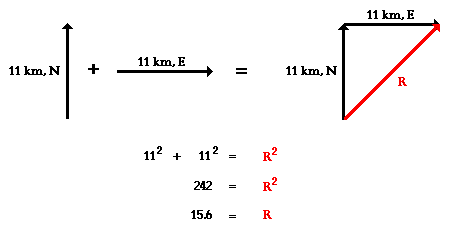# How do vectors work in physics?

##### 1 Answer
Apr 15, 2014

Vectors are lines that represent both magnitude (size) and direction. The length of the vector is drawn to scale, to communicate the size of the measurement; the head of the arrow points in the direction in which the measurement is traveling.

Vectors can be used in a number of ways. Most commonly, they are used to represent displacement or velocity of a moving object, but they can also be used to represent force.

If an object moves in more than one direction subsequently, or if more than one force acts upon an object concurrently, vectors can be added to find a resultant displacement or resultant force. This can be calculated using either a scale diagram and measuring the resultant, or by use of mathematics.For more information, visit http://www.physicsclassroom.com/class/vectors/Lesson-1/Vectors-and-Direction